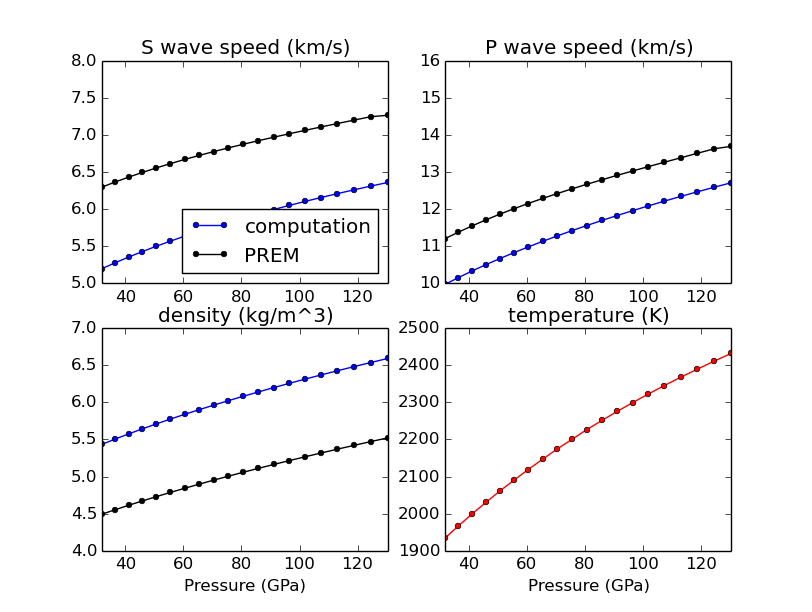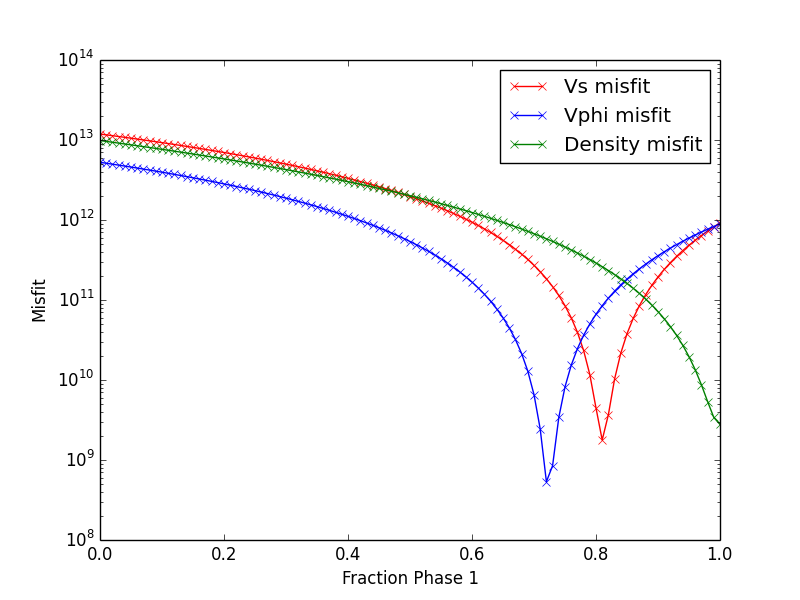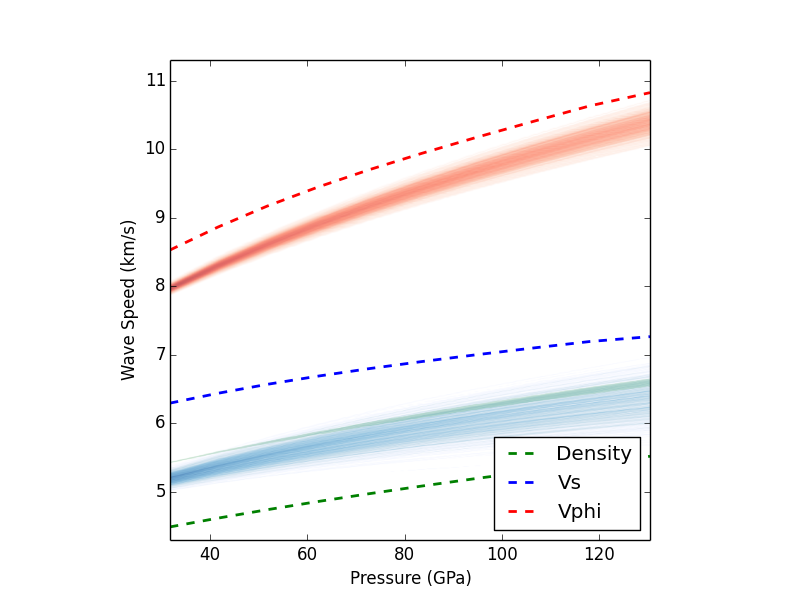# Tutorial¶

The tutorial for BurnMan currently consists of three separate units:

## CIDER 2014 BurnMan Tutorial — step 1¶

In this first part of the tutorial we will acquaint ourselves with a basic script for calculating the elastic properties of a mantle mineralogical model.

In general, there are three portions of this script:

1) Define a set of pressures and temperatures at which we want to calculate elastic properties

2) Setup a composite of minerals (or “rock”) and calculate its elastic properties at those pressures and temperatures.

3) Plot those elastic properties, and compare them to a seismic model, in this case PREM

The script is basically already written, and should run as is by typing:

python step_1.py

on the command line. However, the mineral model for the rock is not very realistic, and you will want to change it to one that is more in accordance with what we think the bulk composition of Earth’s lower mantle is.

When run (without putting in a more realistic composition), the program produces the following image:Your goal in this tutorial is to improve this awful fit...

## CIDER 2014 BurnMan Tutorial — step 2¶

In this second part of the tutorial we try to get a closer fit to our 1D seismic reference model. In the simple Mg, Si, and O model that we used in step 1 there was one free parameter, namely phase_1_fraction, which goes between zero and one.

In this script we want to explore how good of a fit to PREM we can get by varying this fraction. We create a simple function that calculates a misfit between PREM and our mineral model as a function of phase_1_fraction, and then plot this misfit function to try to find a best model.

This script may be run by typing

python step_2.py

Without changing any input, the program should produce the following image showing the misfit as a function of perovskite content:## CIDER 2014 BurnMan Tutorial — step 3¶

In the previous two steps of the tutorial we tried to find a very simple mineralogical model that best fit the 1D seismic model PREM. But we know that there is consideral uncertainty in many of the mineral physical parameters that control how the elastic properties of minerals change with pressure and temperature. In this step we explore how uncertainties in these parameters might affect the conclusions you draw.

The strategy here is to make many different “realizations” of the rock that you determined was the closest fit to PREM, where each realization has its mineral physical parameters perturbed by a small amount, hopefully related to the uncertainty in that parameter. In particular, we will look at how perturbations to $$K_{0}^{'}$$ and $$G_{0}^{'}$$ (the pressure derivatives of the bulk and shear modulus, respectively) change the calculated 1D seismic profiles.

This script may be run by typing

python step_3.py

After changing the standard deviations for $$K_{0}^{'}$$ and $$G_{0}^{'}$$ to 0.2, the following figure of velocities for 1000 realizations is produced: